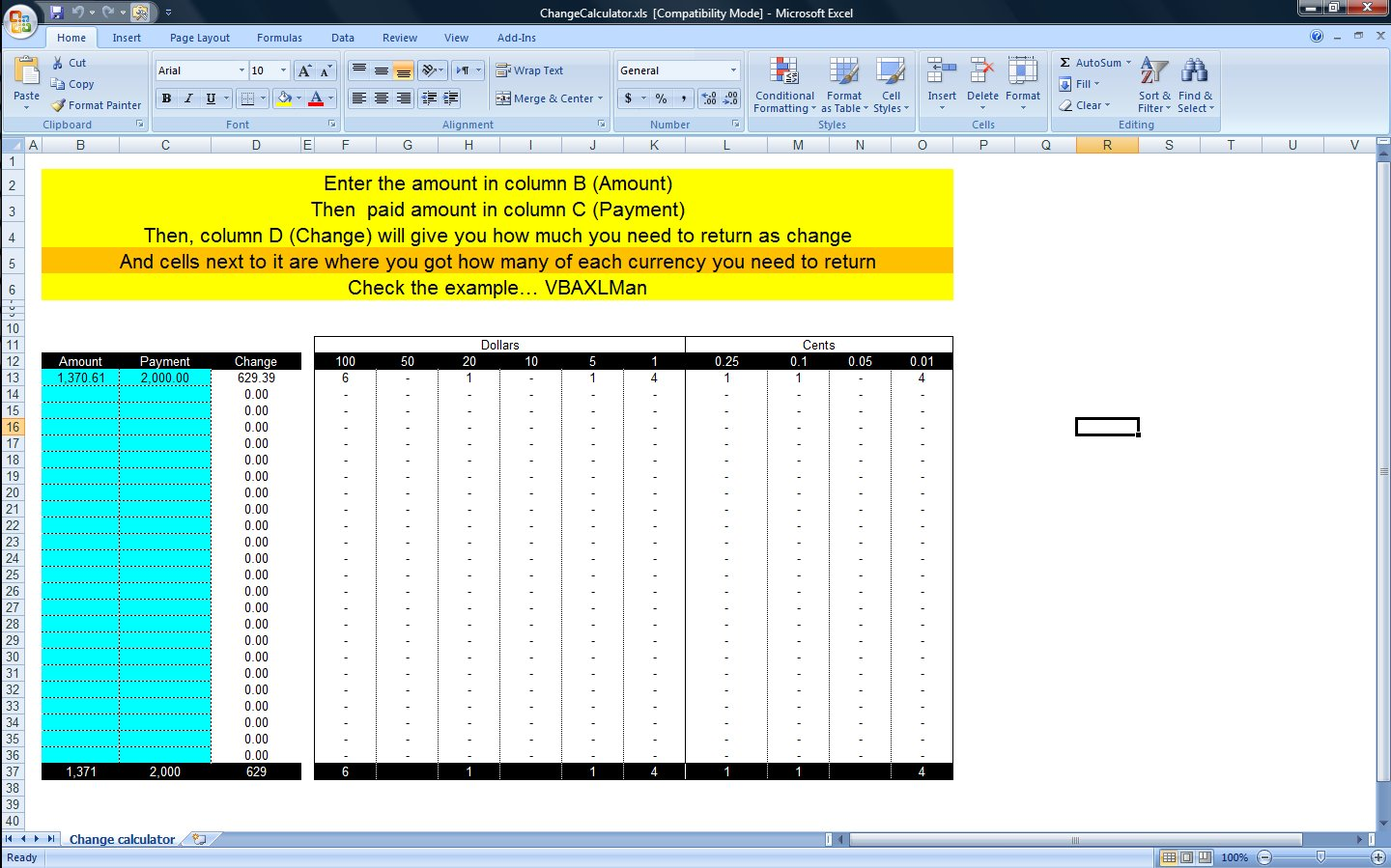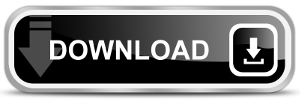# Change Calculator – calculate change in multiple bills/coinsAn Excel file that will determine what cash to return to the buyer (How much of each cash unit, or how many of Fifty dollars and how many of Twenty dollars and so on) in a very interesting way using as less functions as needed
So, if you need to return 15.45, this file will tell you (1 of 10 + 1 of 5 + 4 dims and 1 nickel)

It is very easy way to show that using functions only

Plus, you can change bills/coins and amounts will be updated instantly, try it

It is widely used by Accountants or cashiers to determine what to return to the buyer once you know the amount of the amount and the payments

Formula in column D is:

`=C13-B13`

While columns F through O all have the same formula, which is:

`=IF(INT((\$D13-SUMPRODUCT(\$E13:E13,\$E\$12:E\$12))/F\$12)=0,"-",INT((\$D13-SUMPRODUCT(\$E13:E13,\$E\$12:E\$12))/F\$12))`